# 深度学习（十二）从自编码到栈式自编码

简单的自编码是一种三层神经网络模型，包含了数据输入层、隐藏层、输出重构层，同时它是一种无监督学习模型。在有监督的神经网络中，我们的每个训练样本是（X，y），然后y一般是我们人工标注的数据。比如我们用于手写的字体分类，那么y的取值就是0~9之间数值，最后神经网络设计的时候，网络的输出层是一个10个神经元的网络模型（比如网络输出是(0,0,1,0,0,0,0,0,0,0),那么就表示该样本标签为2）。然而自编码是一种无监督学习模型，我们训练数据本来是没有标签的，那么自编码是这样干的，它令每个样本的标签为y=x，也就是每个样本的数据x的标签也是x。自编码就相当于自己生成标签，而且标签是样本数据本身。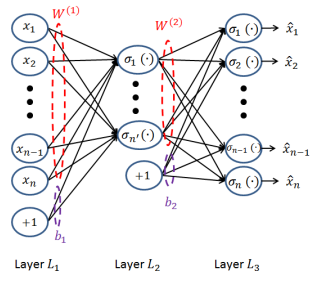(1)从输入层-》隐藏层的原始数据X的编码过程：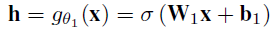(2)从隐藏层-》输出层的解码过程：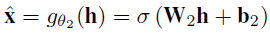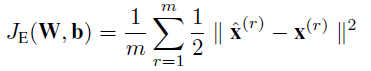当然自编码隐藏层可以比输入层的神经元个数还多，然后我们可以在神经网络的损失函数构造上，加入正则化约束项进行稀疏约束，这个时候就演化成了稀疏自编码了，因此我们接着就说说稀疏自编码。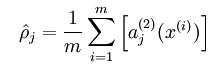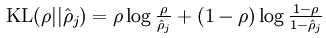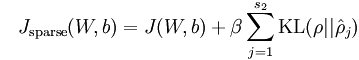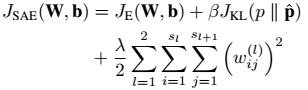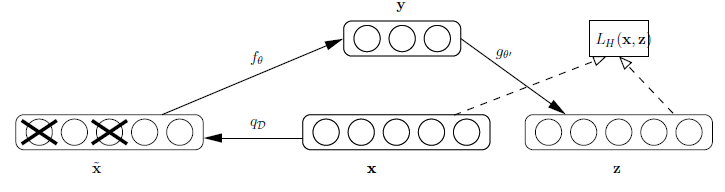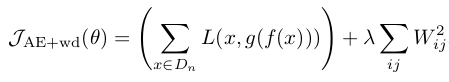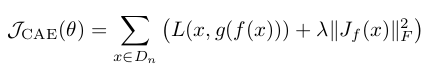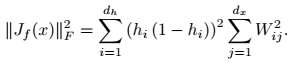h表示隐层神经元。

A、网络各层参数预训练。我们在以前的神经网络中，参数的初始化都是用随机初始化方法，然而这种方法，对于深层网络，在低层中，参数很难被训练，于是Hinton提出了参数预训练，这个主要就是采用RBM、以及我们本篇博文要讲的自编码，对网络的每一层进行参数初始化。也就是我们这边要学的稀疏自编码就是为了对网络的每一层进行参数初始化，仅仅是为了获得初始的参数值而已（这就是所谓的无监督参数初始化，或者称之为“无监督 pre-training”）。

B、比如采用自编码，我们可以把网络从第一层开始自编码训练，在每一层学习到的隐藏特征表示后作为下一层的输入，然后下一层再进行自编码训练，对每层网络的进行逐层无监督训练。

C、当我们无监督训练完毕后，我们要用于某些指定的任务，比如分类，这个时候我们可以用有标签的数据对整个网络的参数继续进行梯度下降调整

OK，我们回到本篇文章的主题，从上面的解释中，我们知道稀疏自编码仅仅只是为了获得参数的初始值而已。栈式自编码神经网络是一个由多层稀疏自编码器组成的神经网络，其前一层自编码器的输出作为其后一层自编码器的输入。栈式自编码就是利用上面所说的：无监督pre-training、有监督微调进行训练训练的深度网络模型。接着就让我们来学一学具体的栈式自编码网络训练。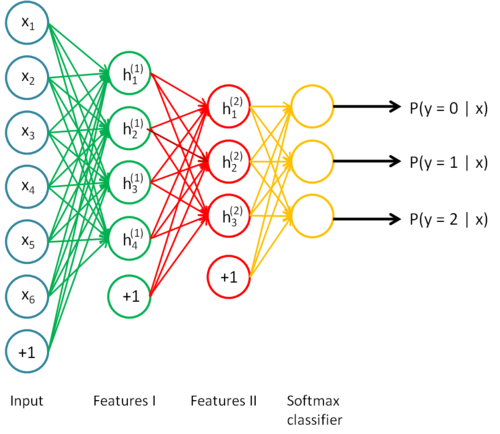1、无监督pre-training阶段

A、首先采用稀疏自编码网络，先训练从输入层到H1层的参数：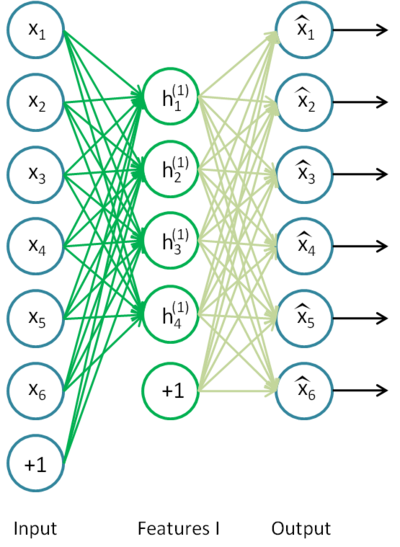B、接着我们训练从H1到H2的参数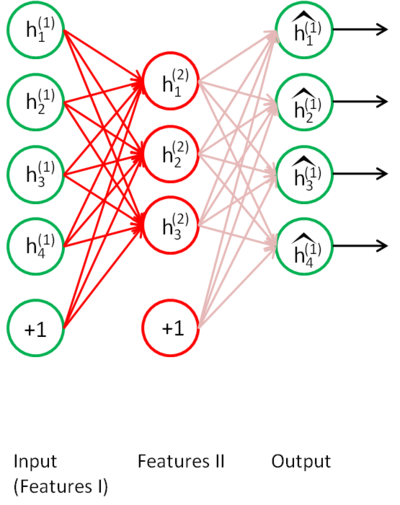C、训练完H2后，我们就可以接分类层softmax，用于多分类任务2、有监督微调阶段

<span style="font-family:Arial;">import os
import sys
import timeit

import numpy

import theano
import theano.tensor as T

from logistic_sgd import load_data
from utils import tile_raster_images

try:
import PIL.Image as Image
except ImportError:
import Image

'''代码编写参考文献：Contractive Auto-Encoder class

class cA(object):

def __init__(self, numpy_rng, input=None, n_visible=784, n_hidden=100,
n_batchsize=1, W=None, bhid=None, bvis=None):
"""
input:输入训练数据数据， input与n_batchsize是对应的，input中有n_batchsize个样本
input每一行代表一个样本，共有n_batchsize个样本

n_visible:可见层神经元的个数

n_hidden: 隐藏层神经元个数

n_batchsize:批量训练，每批数据的个数

W:输入当隐藏层的全连接权值矩阵，因为使用了tied weight 所以从隐藏到输入的权值
矩阵为:W.transpose()

bhid:从输入到隐藏层的偏置单元

bvis:从隐藏层的偏置单元
"""
self.n_visible = n_visible
self.n_hidden = n_hidden
self.n_batchsize = n_batchsize
# 如果没有输入W，则在类里面进行初始化
if not W:
'''W 采用[-a,a]的均匀采样方法进行初始化，因为后面采用s函数，所以
a=4*sqrt(6./(n_visible+n_hidden)) ,矩阵类型theano.config.floatX
这样才能保证在GPU上运行'''
initial_W = numpy.asarray(
numpy_rng.uniform(
low=-4 * numpy.sqrt(6. / (n_hidden + n_visible)),
high=4 * numpy.sqrt(6. / (n_hidden + n_visible)),
size=(n_visible, n_hidden)
),
dtype=theano.config.floatX
)
W = theano.shared(value=initial_W, name='W', borrow=True)

if not bvis:
bvis = theano.shared(value=numpy.zeros(n_visible,
dtype=theano.config.floatX),
borrow=True)

if not bhid:
bhid = theano.shared(value=numpy.zeros(n_hidden,
dtype=theano.config.floatX),
name='b',
borrow=True)

self.W = W
# 输入到隐藏的偏置单元
self.b = bhid
# 隐藏到输出的偏置单元
self.b_prime = bvis
# 使用了tied weights, 所以 W_prime 是 W 的转置
self.W_prime = self.W.T

# 如果没有给定input，那么创建一个
if input is None:
self.x = T.dmatrix(name='input')
else:
self.x = input

self.params = [self.W, self.b, self.b_prime]
#1、输入层到隐藏层
def get_hidden_values(self, input):
return T.nnet.sigmoid(T.dot(input, self.W) + self.b)
#2、隐藏层到输出层。重建结果 x' = s(W' h  + b') ，因为文献使用了tied weigth，所以
#W'等于W的转置，这个可以百度搜索：自编码，tied weight等关键词
def get_reconstructed_input(self, hidden):
return T.nnet.sigmoid(T.dot(hidden, self.W_prime) + self.b_prime)
#计算 J_i = h_i (1 - h_i) * W_i
def get_jacobian(self, hidden, W):
return T.reshape(hidden * (1 - hidden),(self.n_batchsize, 1, self.n_hidden))*T.reshape(W, (1, self.n_visible, self.n_hidden))

#权值更新函数
def get_cost_updates(self, contraction_level, learning_rate):
y = self.get_hidden_values(self.x)#输入-》隐藏
z = self.get_reconstructed_input(y)#隐藏-》输出
J = self.get_jacobian(y, self.W)#y*(1-y)*W
#文献Contractive Auto-Encoders:公式4损失函数计算公式
self.L_rec = - T.sum(self.x * T.log(z) +(1 - self.x) * T.log(1 - z),axis=1)

# 因为J是由n_batchsize*n_hidden计算而来，有n_batchsize个样本，所以要求取样本平均值
self.L_jacob = T.sum(J ** 2) / self.n_batchsize

#整个惩罚函数
cost = T.mean(self.L_rec) + contraction_level * T.mean(self.L_jacob)

#对参数求导
gparams = T.grad(cost, self.params)
#梯度下降法更新参数
updates = []
for param, gparam in zip(self.params, gparams):
updates.append((param, param - learning_rate * gparam))

return (cost, updates)

#测试验证上面的类是否正确
def test_cA(learning_rate=0.01, training_epochs=20,
dataset='mnist.pkl.gz',
batch_size=10, output_folder='cA_plots', contraction_level=.1):
"""
learning_rate:梯度下降法的学习率

training_epochs: 最大迭代次数

contraction_level：为正则项的权重

"""
#datasets为训练集，datasets为验证集，datasets为测试集
datasets = load_data(dataset)
train_set_x, train_set_y = datasets

# 批量下降法，训练的批数
n_train_batches = train_set_x.get_value(borrow=True).shape / batch_size

# allocate symbolic variables for the data
index = T.lscalar()    # 每一批训练数据的索引
x = T.matrix('x')  # 每一批训练数据

if not os.path.isdir(output_folder):
os.makedirs(output_folder)
os.chdir(output_folder)

rng = numpy.random.RandomState(123)

ca = cA(numpy_rng=rng, input=x,
n_visible=28 * 28, n_hidden=500, n_batchsize=batch_size)

cost, updates = ca.get_cost_updates(contraction_level=contraction_level,
learning_rate=learning_rate)
#每一批，训练更新函数，输入参数index
train_ca = theano.function(
[index],
[T.mean(ca.L_rec), ca.L_jacob],
updates=updates,
givens={
x: train_set_x[index * batch_size: (index + 1) * batch_size]
}
)

start_time = timeit.default_timer()

############
# TRAINING #
############

# go through training epochs
for epoch in xrange(training_epochs):
# go through trainng set
c = []
for batch_index in xrange(n_train_batches):
c.append(train_ca(batch_index))

c_array = numpy.vstack(c)
print 'Training epoch %d, reconstruction cost ' % epoch, numpy.mean(
c_array), ' jacobian norm ', numpy.mean(numpy.sqrt(c_array))

end_time = timeit.default_timer()

training_time = (end_time - start_time)

print >> sys.stderr, ('The code for file ' + os.path.split(__file__) +
' ran for %.2fm' % ((training_time) / 60.))
image = Image.fromarray(tile_raster_images(
X=ca.W.get_value(borrow=True).T,
img_shape=(28, 28), tile_shape=(10, 10),
tile_spacing=(1, 1)))

image.save('cae_filters.png')

os.chdir('../')

if __name__ == '__main__':
test_cA()</span>

1、《Contractive Auto-Encoder》

3、Extracting and Composing Robust Features with Denoising Autoencoders

4、《Deep Learning of Part-based Representation of Data Using Sparse Autoencoders with Nonnegativity》

5、《Contractive auto-encoders: Explicit invariance during feature extraction》

**********************作者：hjimce   时间：2015.10.13  联系QQ：1393852684   原创文章，转载请保留原文地址、作者等信息***************